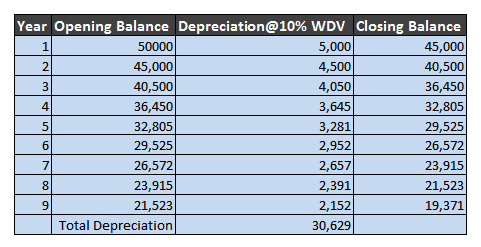###For the sake of quality, our forum is currently "Restricted" to invitation-only. In case if you wish to join our forum, please send an email seeking an invitation to "Contact@Accountingcapital.com".Click on image to update the captcha.

# Scrap Value of an Asset – Meaning

Scrap value of an asset may be defined as the estimated price that can be collected by salvaging or selling the asset after its useful life. In other words, it is the approximated value at which an asset can be sold in the open market after the expiration of its service life.

The residual value of an asset is often insignificant or zero. It is also known as salvage value, residual value or break-up value. Asset disposal account may be created at the time of sale.

Formula to calculate the residual value of an asset is;

 Scrap Value  = (Cost of Asset – Total Depreciation)

Cost of Asset = Purchase Price + Freight + Installation

Scrap value of an asset differs based on the method of depreciation followed by the business .i.e. straight-line method (or) Reducing balance method of depreciation. In the case of fixed assets vs current assets, only the former has “scrap value”.

## Example 1 – Residual Value with the Straight Line Method

Unreal Corp. Ltd purchases machinery worth 50,000 and estimates that its useful life will be 9 years and rate of depreciation (SLM) 10% p.a. So, the given details & calculation is as follows:

Cost of Fixed Asset – 50,000

Estimated life of the asset – 9 years

Depreciation Rate – 10% p.a. i.e. Amt 5,000 p.a. (Total Depreciation = 5,000 x 9)

Salvage value = (50,000 – 45,000) = 5,000

Recommended eLearning Courses & Tutorials

Related Topic – What are NPAs (Non-Performing Assets)?

## Example 2 – Scrap Value with Written Down Value Method

Scenario 2: Assume that the same company in Example 1 follows reducing balance method of depreciation, there will be a variation in the amount of depreciation and scrap value of an asset.

The calculation is as follows:So, the Scrap value = (50,000 – 30,629) = 19,371

If the residual or salvage value of an asset past its useful life is not insignificant it may keep on existing on the company’s balance (asset side) until the time it’s disposed of.